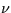Next: 12.7 High-Dispersion Resampled Image Up: 12 Final Archive Data Previous: 12.5 Vector Displacement FITS

12.6 Low-Dispersion Resampled Image FITS File (SILO)

The low-dispersion SI is produced by resampling the photometrically-corrected portion of the low-dispersion LI using the modified Shepard algorithm taken from the Numerical Algorithms Group (NAG) software package. Each pixel is resampled to the position determined by the summation of the vectors computed for:

• shift to photometric correction (ITF) raw space,
• shift from ITF space to geometrically-rectified space,
• rotation such that orders are horizontal,
• wavelength linearization,
• detilting of large-aperture spectra for extended sources only,
• alignment of the apertures for constant wavelength in the line direction,
• adjustment so that both long wavelength cameras provide coverage of the same spectral range,
• adjustment to maintain the spectrum at approximately the same location in the file in the spatial direction,
• adjustment to LWP data to put the large-aperture data at the top of the file, and
• corrections for the spatial deviations (cross-dispersion wiggles) for LWP and LWR data.
The low-dispersion SI is stored in the SILO as a 2-D (640 samples × 80 lines) primary array, with the y coordinate in pixels and the x coordinate in Ångstroms. Each pixel represents a flux number (FN) scaled up by a factor of 32 for storage purposes. The pixels are coded as 16-bit, two's complement integers, with the bits stored in decreasing order of significance. When the image is displayed with the origin in the lower left corner, the large-aperture data appears at the top of the file and the wavelengths increase from left to right. The associatedflags are stored as a SILO image extension, which has the same dimensions as the primary array. Table 12.8 shows the basic FITS keywords for the main header and the image extension header. The starting wavelength and wavelength increment are stored as keywords in the primary header.

Table 12.8:  SILO - Basic FITS Keywords
Keyword and value Description
 SIMPLE = T Standard FITS Format
 BITPIX = 16 16-bits 2's complement pixels
 NAXIS = 2 Two-dimensional image
 NAXIS1 = 640 Dimension along x-axis
 NAXIS2 = 80 Dimension along y-axis
 EXTEND = T Extensions are present
 CRPIX1 = 1. x reference pixel
 CRPIX2 = 1. y reference pixel
 CRVAL1 = nnnn.nn Wavelength at reference pixel
 CRVAL2 = 1. Coordinate of CRPIX2
 CDELT1 = nn.nnnn Increment in wavelengths
 CDELT2 = 1. Increment unit along y-axis
 CTYPE1 = 'WAVELENGTH'  Units along x-axis
 CTYPE2 = 'SCAN '  Units along y-axis
 BUNIT = 'FN '  Flux Numbers
 BSCALE = 3.1250E-02 real=tape*bscale+bzero
 BZERO = 0. Pixel offset
 TELESCOP= 'IUE '  International Ultraviolet Explorer
 FILENAME= 'AAAnnnnn.SILO'  Filename(camera)(number).SILO
 DATE = 'dd/mm/yy'  Date file was written
 ORIGIN = 'VILSPA '  Institution generating the file
 DATAMIN = nnnnn.n  Minimum pixel value
 DATAMAX = nnnnn.n  Maximum pixel value
 XTENSION= 'IMAGE '  Image extension
 BITPIX = 16 16-bit 2's complement pixels
 NAXIS = 2 Two_dimensional image
 NAXIS1 = 640 Dimension along the x-axis
 NAXIS2 = 80 Dimension along the y-axis
 PCOUNT = 0 Number of bytes following data matrix
 GCOUNT = 1 Number of groups
 CRPIX1 = 1. x reference pixel
 CRPIX2 = 1. y reference pixel
 CRVAL1 = nnnn.nn Coordinate of CRPIX1
 CRVAL2 = 1. Coordinate of CRPIX2
 CDELT1 = nn.nnnn Increment unit along the x-axis
 CDELT2 = 1. Increment unit along the y-axis
 CTYPE1 = 'WAVELENGTH'  x-axis units
 CTYPE2 = 'SCAN '  y-axis units
 BUNIT = ' '  Unitless
 FILENAME= 'AAAnnnnn.SFLO'  Filename (camera)(number).SF(disp)
 EXTNAME = 'SILOF '  SILO pixel quality flagsNext: 12.7 High-Dispersion Resampled Image Up: 12 Final Archive Data Previous: 12.5 Vector Displacement FITS
Karen Levay
12/4/1997